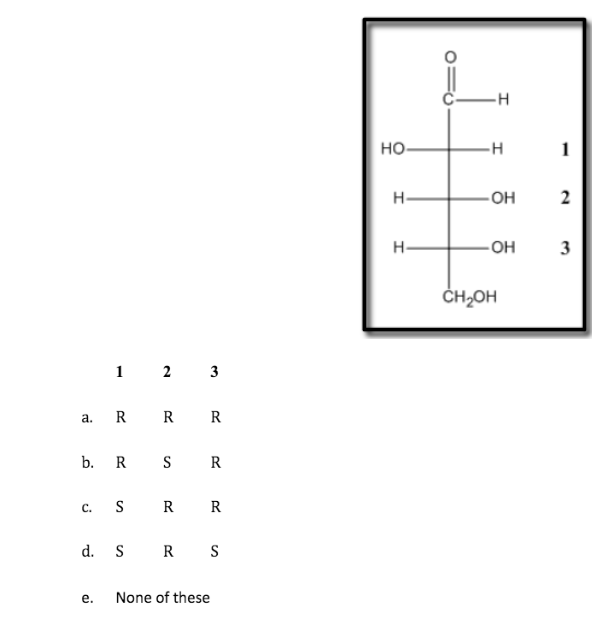# Problem: In the Fischer projection below determine the correct R/S designation for each chirality center [going from top (1) to bottom (3)].

###### Problem Details

In the Fischer projection below determine the correct R/S designation for each chirality center

[going from top (1) to bottom (3)].What scientific concept do you need to know in order to solve this problem?

Our tutors have indicated that to solve this problem you will need to apply the R and S of Fischer Projections concept. You can view video lessons to learn R and S of Fischer Projections. Or if you need more R and S of Fischer Projections practice, you can also practice R and S of Fischer Projections practice problems.

What is the difficulty of this problem?

Our tutors rated the difficulty ofIn the Fischer projection below determine the correct R/S de...as high difficulty.

How long does this problem take to solve?

Our expert Organic tutor, Johnny took 2 minutes and 58 seconds to solve this problem. You can follow their steps in the video explanation above.

What professor is this problem relevant for?

Based on our data, we think this problem is relevant for Professor Lawry's class at BC.